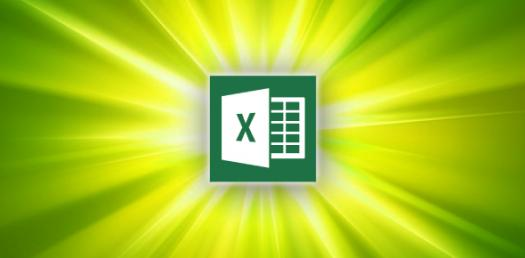# Take The Ultimate Quiz On Microsoft Excel!

19 Questions | Attempts: 976Settings.

• 1.
A fast way to add up this column of numbers is to click in the cell below the numbers and then:
• A.

Click Subtotals on the Data menu.

• B.

View the sum in the formula bar.

• C.

Click the AutoSum button on the Standard toolbar, then press ENTER.

• 2.
Say that you want to paste a formula result — but not the underlying formula — to another cell.You would copy the cell with the formula, then place the insertion point in the cell you want to copy to. What next?
• A.

Click the Paste button on the Standard toolbar.

• B.

Click the arrow on the Paste button on the Standard toolbar, then click Formulas.

• C.

Click the arrow on the Paste button on the Standard toolbar, then click Values.

• 3.
How do you change column width to fit the contents?
• A.

Single-click the boundary to the left of the column heading.

• B.

Double-click the boundary to the right of the column heading.

• C.

Press ALT and single-click anywhere in the column.

• 4.
There are three worksheets with every new workbook. You can change that automatic number if you want to.
• A.

True

• B.

False

• 5.
###### means:
• A.

You've entered a number wrong.

• B.

You've misspelled something.

• C.

The cell is not wide enough.

• 6.
To add a new row, click a cell in the row immediately above where you want the new row.
• A.

True

• B.

False

• 7.
Which key do you press to group two or more nonadjacent worksheets?
• A.

CTRL.

• B.

SHIFT

• C.

ALT

• 8.
Which formula can add the all the numeric values in a range of cells, ignoring those which are not numeric, and place the result in a different cell ?
• A.

Count

• B.

Average

• C.

Sum

• 9.
Is it possible to insert an image from a file into an Excel spreadsheet ?
• A.

Yes

• B.

No

• 10.
Can an Excel spreadsheet be used as the "data source" for a Word Mail Merge ?
• A.

Yes

• B.

No

• 11.
On an Excel sheet the active cell is indicated by ____.
• A.

A dark wide border

• B.

A dotted border

• C.

• D.

None of the above

• 12.
A Formula and a function are the same thing.
• A.

True

• B.

False

• 13.
In order to multiply items in Excel you would use:
• A.

^

• B.

@

• C.

*

• D.

#

• 14.
The formula = ((A2+B5)*5% is valid
• A.

True

• B.

False

• 15.
If cells: A1=90 A2=85 A3=80 A4=75 A5=75 What will be your formula if you are going to get the average?
• 16.
If cells: A1=90 A2=85 A3=80 A4=75 A5=75 What will be your formula if you are going to get the total?
• 17.
How would you Recognize the Cell Address ?
• A.

By Name Box

• B.

By Formula Bar

• C.

By Ribbon

• 18.
A _____________ is the intersection point of a column and a row.
• A.

Column

• B.

Row

• C.

Cell

• 19.
Which sort of a program is the Microsoft Excel 2016 ?
• A.

Word Processing Package.

• B.

Graphics Package

• C.Back to top